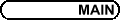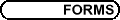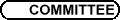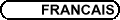Discrete Geometry / Géométrie discrète
Org: Karoly Bezdek (Calgary), Rob Calderbank (Princeton), Robert Connelly (Cornell) and/et Bob Erdahl (Queen's)

KAROLY BEZDEK, University of Calgary, 2500 University Drive NW, Calgary, Alberta T2N 1N4
On the contact graphs of finite unit ball packings in normed spaces

The talk will focus on the problem of the maximum number of touching pairs in a finite packing of translates of a convex body.

TED BISZTRICZKY, University of Calgary
Cyclic polytopes, hyperplanes and codes

In this joint work with K. Boroczky Jr. and D. Gunderson, we consider cyclic d-polytopes P that are realizable with vertices on the d-th order moment curve. A hyperplane H bisects a j-face G of P if H meets the relative interior of G. For k greater than 0, we investigate the maximum number of vertices that P can have so that for some k hyperplanes, each j-face G of P is bisected by one of the hyperplanes. For k greater than 1, the problem translates into the existence of certain codes, or equivalently, certain paths on a k-cube.

AIDEN BRUEN, University of Calgary, 2500 University Drive NW, Calgary, AB T2N 1N4
"The main coding problem"

When sending messages over a noisy channel one introduces redundancy to ensure accurate decoding. After presenting some examples I discuss a version of "The main coding problem" leading to the Singleton bound. Combinatorial applications are surveyed. Linear MDS codes are examined icluding Reed Solomon codes. The foundational work of B. Segre is discussed including his use of the ancient theorem of Menelaus and the Hasse-Weil estimates for algebraic curves in the theory of linear MDS codes. Recent results will be sketched.

ROBERT CONNELLY, Cornell University
The Realizability of Graphs

A graph is d-realizable if, for every configuration of its vertices in EN, there exists a another corresponding configuration in Ed with the same edge lengths. A graph is 2-realizable if and only if it is a partial 2-tree, i.e., a subgraph of the 2-sum of triangles in the sense of graph theory. We show that a graph is 3-realizable if and only if it does not have K5 or the 1-skeleton of the octahedron as a minor.

This is joint work with Maria Sloughter.

ROBERT J. MacG. DAWSON, Saint Mary's University, Halifax, NS B3H 3C3
Crooked Wallpaper

A popular arrangement for rectangular pavers is the "herringbone" pattern. This has the interesting property that its lattice of translational symmetries does not have to have either generator parallel to the edges of the tiles. The "pinwheel" tiling, in which four larger tiles surround a smaller one, also has this property. An obvious application of such tilings is the creation and distribution of "wallpaper" patterns for web pages, desktops, etc., which repeat on a slant, using two rectangular bitmaps.

ROBERT ERDAHL, Queen's University, Kingston, Ontario
Perfect Delaunay Polytopes

We will call a lattice Delaunay polytope D perfect if it has the property that there is a unique circumscribing ellipsoid with interior free of lattice points, and with the surface containing only those lattice points that are the vertices of D. We will call an inhomogeneous quadratic form perfect if it is determined by such a circumscribing "empty ellipsoid". Perfect inhomogeneous forms are associated with perfect Delaunay polytopes in much the way that perfect homogeneous forms are associated with perfect point lattices. We have been able to construct some infinite sequences of perfect Delaunay polytopes, one perfect polytope in each successive dimension starting at some initial dimension; we have been able to construct an infinite number of such infinite sequences. Perfect Delaunay polytopes are intimately related to the theory of Delaunay polytopes, and to Voronois theory of lattice types.

JACOB E. GOODMAN, City College, CUNY, New York, NY 10031
Double-permutation sequences and pseudoline transversals

We describe a new encoding of a family of mutually disjoint compact convex sets in the plane that captures many of its combinatorial properties, and use it to give a new proof of the Edelsbrunner-Sharir theorem that a collection of n compact convex sets in the plane cannot be met by straight lines in more than 2n-2 combinatorially distinct ways. The encoding generalizes the authors' encoding of point configurations by "allowable sequences" of permutations. Since it applies as well to a collection of compact connected sets with a specified pseudoline arrangement A of separators and double tangents, the result extends the Edelsbrunner-Sharir theorem to the case of geometric permutations induced by pseudoline transversals compatible with A.

JOSEPH LING, University of Calgary
A Normed Space with the Beckman-Quarles Property

A classical result of Beckman and Quarles on Euclidean spaces states that a unit-distance preserving function from a Euclidean space of dimension at least 2 into itself is necessarily distance preserving. Since Beckman and Quarles published it in the 1950s, this theorem has been extended in various directions. For what metric spaces are unit-distance preserving functions necessarily distance preserving? This question has yet to receive serious exploration. In this talk, we shall look at an example of a normed space for which the Beckman-Quarles Theorem holds.

BARRY MONSON, University of New Brunswick, Fredericton, NB
Abstract Polytopes over Finite Fields

Any Coxeter group G with string diagram is the symmetry group of an abstract regular polytope (typically infinite). When G is crystallographic, its standard real representation is easily reduced modulo an odd prime p, thus giving a finite representation in some orthogonal space V over Zp. The finite group need not be polytopal; and whether or not it is depends in an intricate way on the geometry of V. Here I outline recent work with Egon Schulte, in which we describe this construction in considerable generality. As a byproduct, we obtain several new families of finite regular maps and even more interesting regular polytopes of higher rank.

ANDREI ORDINE, Queen's University, Kingston, Ontario K7L 3N6
Dual cells in a parallelotope tiling and the theory of designs

Parallelotopes are polytopes in the Euclidean space whose translated copies can be arranged to fill the space so that each two polytopes intersect over a common face, or do not intersect at all. Dirichlet-Voronoi tilings for lattices are examples of parallelotope tilings. It was conjectured by Voronoi that each parallelotope tiling is affinely equivalent to a Dirichlet-Voronoi tiling for some lattice. The conjecture has been proved for a number of special cases, but the general question remains open. The dual tiling to a Dirichlet-Voronoi tiling is called Delaunay tiling.

Dirichlet-Voronoi and Delaunay tilings are of big practical significance in areas ranging from crystallography to numerical solutions of partial differential equations.

Dual cells are analogues of Delaunay polytopes for parallelotope tilings where it is not known whether the tiling is affinely equivalent to a Dirichlet-Voronoi tiling for some lattice. In my study of parallelotope tilings, I came across the following problem.

Find all dual 4-cells which contain a system R of dual 2-cells, so that all dual 2-cells in R are parallelograms, and the following conditions hold:

1. Each two parallelograms in R interest over a common vertex and span a 4-dimensional affine space,

2. Each vertex of a parallelogram in R belongs to at least one other parallelogram in R.

The answer is that there are no such dual 4-cells.

Quite unexpectedly, this problem turned out to be connected to graph theory and the theory of designs. The talk will show the origin of the problem, and the way it was solved.

KOSTYA RYBNIKOV, University of Massachusetts Lowell, 1 University Ave., MATH Sciences, Lowell, MA 01854
General Convexity Theory

Many concepts of convexity theory are of topological nature, yet they are usually developed with significant involvement of linear algebra and analysis. This traditional approach is unsatisfactory when we need to generalize results of convexity theory to spaces without differential or vector structure.

I suggest a rather general notion of topological space with a convexity structure. The notion of (non-unique) convex hull plays the major role in this generalization. The resulting degree of generality allows for treatment of spaces where geodesic segments are not unique even locally, like, e.g., in the case of PL-surfaces.

Although the suggested axiom system is very general, it admits rather strong and useful theorems such as our generalization of a well-known theorem of Klee on the face-structure of the boundary of a convex set in the Euclidean space.

NORBERT SAUER, University of Calgary, 2500 University Drive NW, Calgary, Alberta
Families of graphs

Given objects and a notion of morphism, we study families F of objects defined by:

 F : = {A : for all i Î I (Bi\not® A)}
where (Bi; i Î I) is a set of objects, often just a singleton set or a finite list of objects.

In this talk we use as an example finite graphs and the category of induced embeddings.

Given graphs F1, F2, ..., Fn let:

 Forb (F1, F2, ..., Fn) : = {graphs G : Fi \not® G for all 1 £ i £ n}.

In this talk we discuss the connection between partition properties, chromatic numbers and amalgamation of graphs in such families Forb (F1, F2, ..., Fn) of graphs.

EGON SCHULTE, Northeastern University, Department of Mathematics, Boston, MA 02115, USA
Combinatorial Tiling and Symmetry

A locally finite face-to-face tiling T of euclidean d-space Ed is monotypic if each tile is a convex d-polytope combinatorially equivalent to a given polytope, the combinatorial prototile of T. Two problems about combinatorial symmetry of monotypic tilings are addressed. In particular, we present the Local Theorem for Monotypic Tilings, which describes a local characterization of combinatorial tile-transitivity of monotypic tilings in Ed; this is joint work with Nikolai Dolbilin. Moreover, we discuss a combinatorial analogue of aperiodicity of prototile sets.

BRIGITTE SERVATIUS, WPI, Worcester, MA 01609-2280, USA
The 2-Dimensional Rigidity of Certain Families of Graphs

We investigate how graph theoretic properties such as regularity, planarity, and transitivity influence planar rigidity and apply some of these results to reveal rigidity properties of random graphs. We show that random d-regular graphs are generically globally rigid in the plane for all d ³ 4.

MARIA SLOUGHTER, Cornell University
Realizability of graphs

A graph is d-realizable if, for every realization of the graph in some Euclidean space, there exists a realization in d-dimensional Euclidean space with the same edge lengths. For example, any tree is 1-realizable, but a triangle is not. In this talk, we will classify all 3-realizable graphs. In particular, we will show that two specific graphs V8 and C5 ×C2 are 3-realizable.

JOZSEF SOLYMOSI, University of British Columbia, Vancouver, Canada
Lines and Cartesian products on the Complex Plane

Let Ta,b denote the transformation az+b on the complex numbers. Given a finite set of complex numbers, A, we give a tight bound on the number of transformations such that |Ta,b(A)ÇA| ³ k. As an application, we prove the following statement. For any point set P={p1,p2,...,pt} and another set A={a1,a2,...,an} on the plane, the number of those subsets of A which are similar to P is bounded by cn2/t, where c is a universal constant, independent from n and t.

ILEANA STREINU, Smith College, Northampton

GODFRIED TOUSSAINT, McGill
On Constructing a Polyhedron from its Vertex Set

Given a set S of n ³ 3 points in the plane (not all on a line) it is well known that it is always possible to construct a simple polygon P such that the vertices of P are precisely the given points in S. For example, the shortest circuit through S must be such a simple polygon. In 1994 Grünbaum showed that an analogous theorem holds in 3-dimensional space. More precisely, if S is a set of n ³ 4 points in space (not all of which are coplanar) then it is always possible to construct a simple (sphere-like) polyhedron P such that the vertices of P are precisely the given points in S. Grünbaum's constructive proof may yield Schonhardt polyhedra that cannot be triangulated. In this talk several alternative algorithms are reviewed for constructing such polyhedra induced by a set of points, which may always be triangulated, and which enjoy several other useful properties as well. Such properties include polyhedra that are star-shaped, have hamiltonian skeletons, and admit efficient point location queries. Efficient algorithms for constructing such polyhedra will be described.

ASIA IVIC WEISS, York University, 4700 Keele Street, Toronto, Ontario
Self-duality of chiral polytopes

We prove that self-dual chiral polytopes of odd rank posses a polarity, that is, an involutory duality, and give an example showing this is not true in even ranks. Properties of the extended groups of automorphisms and dualities, of self-dual chiral polytopes are discussed.

SUE WHITESIDES, McGill University, 318-3480 University St., Montreal, Quebec H3A 2A7
Hardness of a 3D Graph Embedding Problem

We consider the following graph embedding question: given a graph G, is it possible to map its vertices to points in 3D such that G is isomorphic to the mutual nearest neighbor graph of the set P of points to which the vertices are mapped? We show that this problem is NP-hard. We do this by extending the "logic engine" method to three dimensions by using building blocks inspired by the structure of diamond and by constructions of Alexander Graham Bell and Buckminster Fuller.

Joint work with Matthew Kitching.

ALLAN R. WILKS, AT&T Labs - Research, 180 Park Avenue, Florham Park, NJ 07932-0971